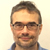# Whole R value 2×8 vs 2×6| Posted in Energy Efficiency and Durability on

I have been studying up on the building science High R value wall study and am trying to wrap my head around why the whole R value of a 2×8 wall appears to get a much bigger penalty when it comes to whole R value than a 2×6 wall. I was thinking must be extra thermal bridging somehow, but even if you start throwing XPS on the outside it doesn’t seem to change things much. What am I missing here

Figures from BuildingScience High R values Report

2×8 advanced frame wall with R31 blown in fiberglass
R31 insulated value
R22.2 whole R value
Whole R value difference R8.8 or 28%

2×6 advanced frame wall with R21 fiberglass batt
R21 insulated value
R17.2 whole R value
Whole R difference R3.8 or 18%

2×8 AF wall with R31 fiberglass + 1″XPS
R36 insulated value
R27.2 whole R value
Whole R value difference R8.8 or 24%

2×6 AF wall with R21 fiberglas + 1″ XPS
R26 insulated value
R22.2 whole R value
Whole R value difference R3.8 or 14.6%## Join the leading community of building science experts

### Replies

1.Expert Member
| | #1

The framing fraction used in the calculations was 19% in all cases.

The framing fraction of 2x8 framing has an R value of about R1.2/inch x 7.5" = R9.

The framing fraction of 2x6 framing has an R value of abou tR1.2/inch x 5.5" = R6.6.

If you add R5 of foam to the exterior of the 2 x 6 framing it cuts the framing loss by about 45%. Adding it to 2x8 framing it cuts it only about 35%

R21/.R6.6= 3.18, which means R6.6 loses 3.18 x as much heat per square foot as R21. So the un-dimensioned heat transfer through the 19% framing fraction is 19 x 3.18= 60 to the cavity fill's 81. (19% +81%=100%). That means the heat transfer of the framing fraction is 60/81= ~75% that of the insulated fraction, or about 43% of the total heat transfer.

R31/R9= 3.44, so the raming fraction of the 2x8 loses 3.44 x as much per square foot. The un-dimensioned heat transfer through the 19% framing fraction is then 19 x 3.44= 65, to the insulated portion's 81. Thus the framing fraction transfers 65 / 81= ~80% of the amount of heat that the insulated portion is passing. That means the framing fraction is transfering 44% of th the heat going through the framing & insulation layer.

For the crude 2-D model good enough for most purposes:

R=1/U

Rframing = 1.2 x depth in inches

R-insulation= the labeled R

Rgypsum= 0.5

Rsheathing = 0.5.

The average R of the framing + insulation layer:

1/ Uaverage

Uaverage for the 19% framing fraction is 0.19/Rframing + 0.81/R insulation

eg for the 2x6 section, 0.19/R6.6 + 0.81/ R21= 1.41/R21

So the R-value of the framing & insulation layer is 1/ (1.41/R21)= R14.9

For the whole-wall R you add R0.5 gypsum + R0.5 sheathing to R14.9 to get roughly R15.9.

That's not a perfect match to the R17.2 they came up using THERM, but it's good 'nuff for the kind o' girlz I hang with, being only 7% short of the better model. :-)

They may have used different assumptions about the R-value of the siding, and added in the interior & exterior air films to end up at R17.2, which would be legit.

For heat load calculation purposes the U-factor of R15.9 whole wall is U0.063, for R17.2 it's 0.058.

Rounding either to U0.06, since the difference is truly "in the noise" of construction error, and minor defects, and the actual R-values of the materials vs. labeled R values. The implied precision of that many significant digits is silly in real-world assemblies.

2.| | #2

Thanks so much Dana - that makes sense and is very helpful.

For the exterior foam scenarios where do you add that R value to the formula to factor in the minimizing of the thermal bridging. You mentioned that in 2x6 R5 cuts framing loss by 45% and only 35% in 2x8, but wasn't sure how/where to calculate that with different levels of external rigid insulation.

• |
• |
• |
• |